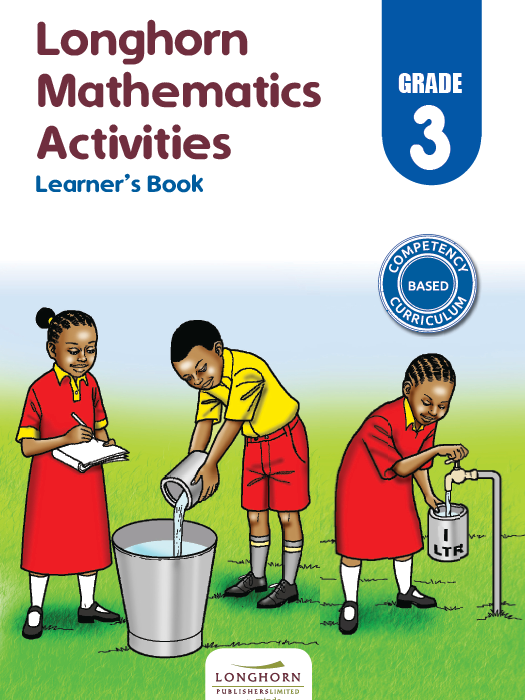Study kit (Math 3)##### Authors
T. Mukhuri, M. Okello, M. Mwimani, J. Mwaniki, S. Ndinwa
##### Exercises kit’s authors
T. Mukhuri, M. Okello, M. Mwimani, J. Mwaniki, S. Ndinwa
##### Publisher
Longhorn Publishers
##### Included in packages• The study kit contains 101 chapters and teacher’s exercises kit contains 106 exercises.
• ##### Authors
T. Mukhuri, M. Okello, M. Mwimani, J. Mwaniki, S. Ndinwa
• ##### Exercises kit’s authors
T. Mukhuri, M. Okello, M. Mwimani, J. Mwaniki, S. Ndinwa
Mathematics
English
• ##### Publisher
Longhorn Publishers

### 1. Number concept

##### Chapter
1.1. Preparing ourselves
Free chapter!
1.2. Ordinal numbers up to tenth (10th)
1.3. Ordinal numbers up to twentieth (20th)

### 2. Whole numbers

##### Chapter
2.2. Writing numbers 1 to 1000 in symbols
2.3. Place value (1)
2.4. Place value (2)
2.5. Value of a digit in a number
2.6. Reading and writing numbers in words
2.7. Identifying missing numbers in number patterns
2.8. Appreciating number patterns
2.9. Digital games

### 3. Fractions

##### Chapter
3.1. Half of a whole
3.2. A quarter of a whole
3.3. One eighth of a whole
3.4. Half of a group
3.5. Quarter of a group
3.6. An eighth of a group

##### Chapter
4.1. Horizontal addition of 3 and 2-digit numbers without regrouping
4.2. Vertical addition of 3 and 2-digit numbers without regrouping
4.3. Horizontal addition of 3 and 2-digit numbers with regrouping
4.4. Vertical addition of 3 and 2-digit numbers with regrouping
4.5. Addition of three 1-digit number by counting on
4.6. Addition of three 1-digit number using a number line
4.7. Horizontal addition two 3-digit number without regrouping
4.8. Vertical addition two 3-digit number without regrouping
4.9. Horizontal addition two 3-digit number with single regrouping
4.10. Vertical addition two 3-digit number with single regrouping
4.11. Digital game

### 5. Subtraction

##### Chapter
5.1. Horizontal subtraction of 2-digit numbers from 3-digit numbers without regrouping
5.2. Vertical subtraction of 2-digit numbers from 3-digit numbers without regrouping
5.3. Horizontal subtraction of 2-digit numbers from 3-digit numbers with regrouping
5.4. Vertical subtraction of 2-digit numbers from 3-digit numbers with regrouping
5.5. Horizontal subtraction of two 3-digit numbers without regrouping
5.6. Vertical subtraction of two 3-digit numbers without regrouping
5.7. Horizontal subtraction of two 3-digit numbers with regrouping
5.8. Vertical subtraction of two 3-digit numbers with regrouping
5.9. Word problems on subtraction
5.10. Finding missing numbers in subtraction sentences
5.11. Number Patterns
5.12. Digital game

### 6. Multiplication

##### Chapter
6.1. Multiplication by 1
6.2. Multiplication by 2
6.3. Multiplication by 3
6.4. Multiplication by 4
6.5. Multiplication by 5
6.6. Multiplication by 6
6.7. Multiplication by 7
6.8. Multiplication by 8
6.9. Multiplication by 9
6.10. Multiplication by 10
6.11. Multiplication table

### 7. Division

##### Chapter
7.1. Division as equal sharing
7.2. Division as repeated subtraction
7.3. Relationship between division and multiplication
7.4. Division and multiplication using the multiplication table
7.5. Multiplication and division by 10

### 8. Length

##### Chapter
8.1. Measuring length in metres
8.2. Addition of length in metres
8.3. Word problems involving addition of lengths
8.4. Subtraction of length in metres
8.5. Word problems involving subtraction of length
8.6. Estimating length

### 9. Mass

##### Chapter
9.1. Measuring mass in kilogrammes
9.2. Addition of mass in real life
9.3. Subtraction of mass in real-life
9.4. Estimating mass

### 10. Capacity

##### Chapter
10.1. Measuring capacity in litres
10.2. Addition of capacity in litres
10.3. Word problems involving addition of capacity
10.4. Subtraction of capacity in litres
10.5. Word problems involving substraction of capacity
10.6. Estimating capacity

### 11. Time

##### Chapter
11.1. Clocks and watches
11.2. Minute as unit of measuring time
11.3. Relationship between hours and minutes
11.4. Telling time in quarter past
11.5. Telling time in half past
11.6. Telling time in quarter to
11.7. Telling time in minutes past or to an hour
11.8. Telling time using digital watches
11.9. Estimating time in hours
11.11. Subtraction involving time

### 12. Money

##### Chapter
12.1. Kenyan currency
12.2. Counting money in different denominations
12.4. Subtraction of money
12.5. Shopping activities
12.6. Change
12.7. Needs and wants
12.8. Appreciating spending and saving money

### 13. Position and direction

##### Chapter
13.1. Position and direction
13.2. Digital game